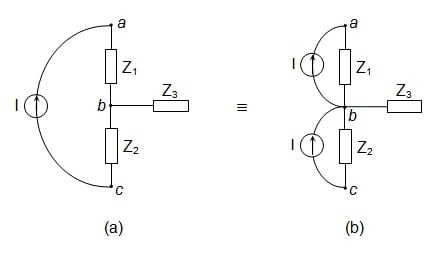# Mesh current analysis question

I am able to solve the question by transforming the original circuit into an equivalent circuit. However, I cannot seem to solve the question using mesh current analysis on the original circuit diagram.• $i_3 \ne\small 0.2\:A$ – Chu Jul 13 '18 at 13:03
• ... loop current method can't normally be used with current sources - use KCL/KVL mesh analysis or node analysis. – Chu Jul 13 '18 at 13:24
• I am able to solve the question using node voltage analysis. And I thought that if there is a current source present, it forces the mesh current to be equal to the value of the current source. Do you mind enlightening me on what is a KCL/KVL mesh analysis? I am not familiar with that method. – Tom Tom Jul 13 '18 at 13:40
• Look up 'branch current method' rather than 'loop current method'. In the former method currents are placed on conductors according to Kirchhoff's current law (KCL). When you solve for the loop voltages, do not choose loops that include current sources. – Chu Jul 13 '18 at 13:45

When using the mesh current method you are essentially writing KVL equations around each of the meshes. Each term in the equation is a voltage term, either an $I \times R$ term or an actual voltage (if the mesh has a voltage source).

Current sources can be a problem because you can't write a term for the voltage across the source. When there is a current source that is on an outer edge of the circuit then that current source is only part of a single mesh and you get the mesh current by inspection.

When a current source is shared by two meshes then you have to create a supermesh that combines the two meshes but excludes the current source. Then you can write, by inspection, an equation that specifies that the difference between the two mesh currents is equal to the current source value.

We don't do homework problems here so I'm just giving you some hints.

You can also consider using source shifting to get rid of the current source:Both are equivalent from an analysis point of view. After doing this step, you can use Thevenin equivalents to turn the current sources into voltage sources.